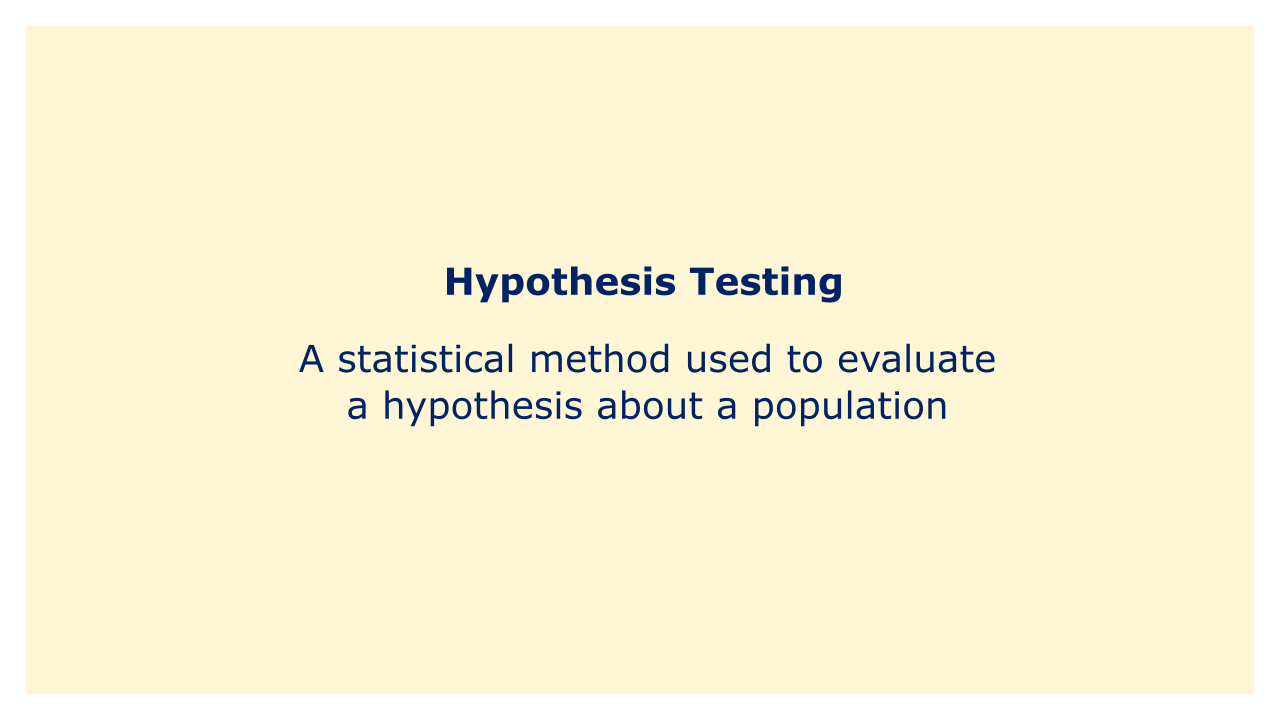# Hypothesis TestingImage: Moneybestpal.com

### A statistical technique called hypothesis testing is used to assess a population-based hypothesis. Hypothesis testing is a technique used in finance to examine whether or not the evidence supports a particular hypothesis about a particular financial market or product.

A null hypothesis must be established, which states that there is no significant difference between any two groups or variables.

The next step is to create an alternative hypothesis, which is a claim that disputes the null hypothesis. The null hypothesis can then be rejected in favor of the alternative hypothesis by collecting and analyzing the evidence using statistical techniques.

The likelihood of making a type I error, or rejecting the null hypothesis when it is actually true, is used as the significance threshold when doing hypothesis testing. Based on the data, a p-value is calculated and put up against the significance level. The alternative hypothesis is accepted in place of the null hypothesis if the p-value is less than the significance level.

In finance, hypothesis testing is frequently used to assess the effectiveness of investment strategies, gauge market efficiency, and establish if asset returns display a particular distribution or pattern.
Tags